Students can download Maths Chapter 5 Coordinate Geometry Ex 5.2 Questions and Answers, Notes, Samacheer Kalvi 10th Maths Guide Pdf helps you to revise the complete Tamilnadu State Board New Syllabus, helps students complete homework assignments and to score high marks in board exams.

## Tamilnadu Samacheer Kalvi 10th Maths Solutions Chapter 5 Coordinate Geometry Ex 5.2

Question 1.
What is the slope of a line whose inclination with positive direction of x -axis is
(i) 90°
(ii) 0°
Solution:
Here θ = 90°
Slope (m) = tan θ
Slope = tan 90°
= undefined.

(ii) Here θ = 0°
Slope (m) = tan θ
Slope = tan 0°
= 0Question 2.
What is the inclination of a line whose slope is
(i) 0
(ii) 1
Solution:
(i) m = 0
tan θ = 0 ⇒ θ = 0°
(ii) m = 1 ⇒ tan θ = tan 45° ⇒ 0 = 45°

Question 3.
Find the slope of a line joining the points
(i) (5,$$\sqrt { 5 }$$) with the origin
(ii) (sin θ, -cos θ) and (-sin θ, cos θ)
Solution:
(i) The given points is (5,$$\sqrt { 5 }$$) and (0, 0)
Slope of a line = $$\frac{y_{2}-y_{1}}{x_{2}-x_{1}}$$ = $$\frac{0-\sqrt{5}}{0-5}$$
= $$\frac{\sqrt{5}}{5}=\frac{1}{\sqrt{5}}$$

(ii) The given points is (sin θ, -cos θ) and (-sin θ, cos θ)
Slope of a line = $$\frac{y_{2}-y_{1}}{x_{2}-x_{1}}=\frac{\cos \theta+\cos \theta}{-\sin \theta-\sin \theta}$$
= $$\frac{2 \cos \theta}{-2 \sin \theta}$$ = – cot θ

Question 4.
What is the slope of a line perpendicular to the line joining A(5,1) and P where P is the mid-point of the segment joining (4,2) and (-6,4).
Solution:
Mid point of XY = $$\left(\frac{x_{1}+x_{2}}{2}, \frac{y_{1}+y_{2}}{2}\right)$$ = ($$\frac { 4-6 }{ 2 }$$,$$\frac { 2+4 }{ 2 }$$)
= ($$\frac { -2 }{ 2 }$$,$$\frac { 6 }{ 2 }$$) = (-1, 3)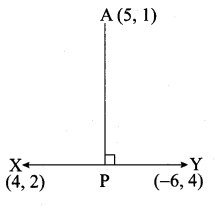Slope of a line = $$\frac{y_{2}-y_{1}}{x_{2}-x_{1}}$$ = ($$\frac { 3-1 }{ -1-5 }$$)
= $$\frac { 2 }{ -6 }$$ = – $$\frac { 1 }{ 3 }$$Question 5.
Show that the given points are collinear: (-3, -4), (7,2) and (12, 5)
Solution:
The vertices are A(-3, -4), B(7, 2) and C(12, 5)
Slope of a line = $$\frac{y_{2}-y_{1}}{x_{2}-x_{1}}$$
Slope of AB = $$\frac { 2+4 }{ 7+3 }$$ = $$\frac { 6 }{ 10 }$$ = $$\frac { 3 }{ 5 }$$
Slope of BC = $$\frac { 5-2 }{ 12-7 }$$ = $$\frac { 3 }{ 5 }$$
Slope of AB = Slope of BC = $$\frac { 3 }{ 5 }$$
∴ The three points A,B,C are collinear.

Question 6.
If the three points (3, -1), (a, 3) and (1, -3) are collinear, find the value of a.
Solution:
The vertices are A(3, -1), B(a, 3) and C(1, -3)
Slope of a line = $$\frac{y_{2}-y_{1}}{x_{2}-x_{1}}$$
Slope of AB = $$\frac { 3+1 }{ a-3 }$$ = $$\frac { 4 }{ a-3 }$$
Slope of BC = $$\frac { 3+3 }{ a-1 }$$ = $$\frac { 6 }{ a-1 }$$
Since the three points are collinear.
Slope of AB = Slope BC
$$\frac { 4 }{ a-3 }$$ = $$\frac { 6 }{ a-1 }$$
6 (a – 3) = 4 (a – 1)
6a – 18 = 4a – 4
6a – 4a = -4 + 18
2a = 14 ⇒ a = $$\frac { 14 }{ 2 }$$ = 7
The value of a = 7Question 7.
The line through the points (-2, a) and (9,3) has slope –$$\frac { 1 }{ 2 }$$ Find the value of a.
Solution:
The given points are (-2, a) and (9, 3)
Slope of a line = $$\frac{y_{2}-y_{1}}{x_{2}-x_{1}}$$
– $$\frac { 1 }{ 2 }$$ = $$\frac { 3-a }{ 9+2 }$$ ⇒ – $$\frac { 1 }{ 2 }$$ = $$\frac { 3-a }{ 11 }$$
2(3 – a) = -11 ⇒ 6 – 2a = -11
-2a = -11 – 6 ⇒ -2a = -17 ⇒ a = – $$\frac { 17 }{ 2 }$$
∴ The value of a = $$\frac { 17 }{ 2 }$$

Question 8.
The line through the points (-2, 6) and (4, 8) is perpendicular to the line through the points (8,12) and (x, 24). Find the value of x.
Solution:
Find the slope of the line joining the point (-2, 6) and (4, 8)
Slope of line (m1) = $$\frac{y_{2}-y_{1}}{x_{2}-x_{1}}$$
= $$\frac { 8-6 }{ 4+2 }$$ = $$\frac { 2 }{ 6 }$$ = $$\frac { 1 }{ 3 }$$
Find the slope of the line joining the points (8, 12) and (x, 24)
Slope of a line (m2) = $$\frac { 24-12 }{ x-8 }$$ = $$\frac { 12 }{ x-8 }$$
Since the two lines are perpendicular.
m1 × m2 = -1
$$\frac { 1 }{ 3 }$$ × $$\frac { 12 }{ x-8 }$$ = -1 ⇒ $$\frac{12}{3(x-8)}=-1$$
-1 × 3 (x – 8) = 12
-3x + 24 = 12 ⇒ – 3x = 12 -24
-3x = -12 ⇒ x = $$\frac { 12 }{ 3 }$$ = 4
∴ The value of x = 4Question 9.
Show that the given points form a right angled triangle and check whether they satisfies Pythagoras theorem.
(i) A(1, -4) , B(2, -3) and C(4, -7)
(ii) L(0, 5), M(9,12) and N(3,14)
Solution:
(i) The vertices are A(1, -4), B(2, -3) and C(4, -7)
Slope of a line = $$\frac{y_{2}-y_{1}}{x_{2}-x_{1}}$$
Slope of AB = $$\frac { -3+4 }{ 2-1 }$$ = $$\frac { 1 }{ 1 }$$ = 1
Slope of BC = $$\frac { -7+3 }{ 4-2 }$$ = $$\frac { -4 }{ 2 }$$ = -2
Slope of AC = $$\frac { -7+4 }{ 4-1 }$$ = – $$\frac { 3 }{ 3 }$$ = -1
Slope of AB × Slope of AC = 1 × -1 = -1∴ AB is ⊥r to AC
∠A = 90°
∴ ABC is a right angle triangle
Verification: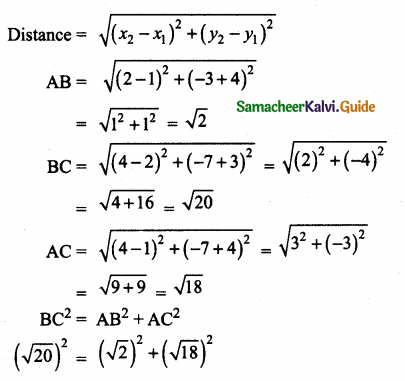20 = 2 + 18
20 = 20 ⇒ Pythagoras theorem verified(ii) The vertices are L(0, 5), M(9, 12) and N(3, 14)
Slope of a line = $$\frac{y_{2}-y_{1}}{x_{2}-x_{1}}$$
Slope of LM = $$\frac { 12-5 }{ 9-0 }$$ = $$\frac { 7 }{ 9 }$$Slope of MN = $$\frac { 14-12 }{ 3-9 }$$ = $$\frac { 2 }{ -6 }$$ = – $$\frac { 1 }{ 3 }$$
Slope of LN = $$\frac { 14-5 }{ 3-0 }$$ = $$\frac { 9 }{ 3 }$$ = 3
Slope of MN × Slope of LN = – $$\frac { 1 }{ 3 }$$ × 3 = -1
∴ MN ⊥ LN
∠N = 90°
∴ LMN is a right angle triangle
Verification: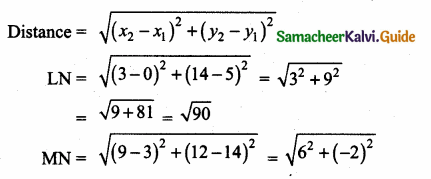130 = 90 + 40
130 = 130 ⇒ Pythagoras theorem is verifiedQuestion 10.
Show that the given points form a parallelogram:
A (2.5,3.5), B(10, -4), C(2.5, -2.5) and D(-5, 5).
Solution:
Let A(2.5, 3.5), B(10, -4), C(2.5, -2.5) and D(-5, 5) are the vertices of a parallelogram.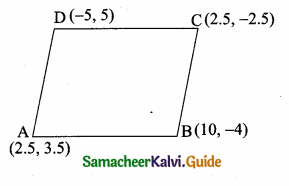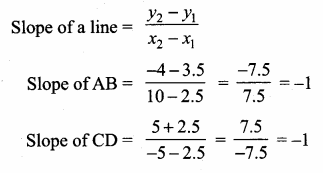Slope of AB = Slope of CD = -1
∴ AB is Parallel to CD ……(1)Slope of BC = Slope of AD
∴ BC is parallel to AD
From (1) and (2) we get ABCD is a parallelogram.Question 11.
If the points A(2, 2), B(-2, -3), C(1, -3) and D(x, y) form a parallelogram then find the value of x and y.
Solution:
Let A(2, 2), B(-2, -3), C(1, -3) and D(x, y) are the vertices of a parallelogram.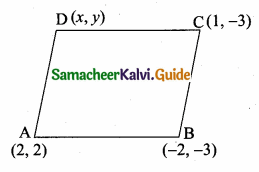Slope of a line = $$\frac{y_{2}-y_{1}}{x_{2}-x_{1}}$$
Slope of AB = $$\frac { -3-2 }{ -2-2 }$$ = $$\frac { -5 }{ -4 }$$ = $$\frac { 5 }{ 4 }$$
Slope of BC = $$\frac { -3+3 }{ -2-1 }$$ = $$\frac { 0 }{ -3 }$$ = 0
Slope of CD = $$\frac { y+3 }{ x-1 }$$
Slope of AD = $$\frac { y-2 }{ x-2 }$$
Since ABCD is a parallelogram
Slope of AB = Slope of CD
$$\frac { 5 }{ 4 }$$ = $$\frac { y+3 }{ x-1 }$$
5(x – 1) = 4 (y + 3)
5x – 5 = 4y + 12
5x – 4y = 12 + 5
5x – 4y = 17 ……(1)
Slope of BC = Slope of AD
0 = $$\frac { y-2 }{ x-2 }$$
y – 2 = 0
y = 2
Substitute the value of y = 2 in (1)
5x – 4(2) = 17
5x -8 = 17 ⇒ 5x = 17 + 18
5x = 25 ⇒ x = $$\frac { 25 }{ 5 }$$ = 5
The value of x = 5 and y = 2.Question 12.
Let A(3, -4), B(9, -4) , C(5, -7) and D(7, -7). Show that ABCD is a trapezium.
Solution:
Let A(3, -4), B(9, -4), C(5, -7) and D(7, -7) are the vertices of a quadrilateral.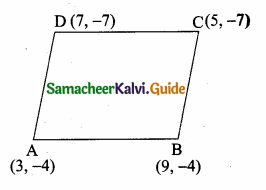Slope of a line = $$\frac{y_{2}-y_{1}}{x_{2}-x_{1}}$$
Slope of AB = $$\frac { -4+4 }{ 9-3 }$$ = $$\frac { 0 }{ 6 }$$ = 0
Slope of BC = $$\frac { -7+4 }{ 5-9 }$$ = $$\frac { -3 }{ -4 }$$ = $$\frac { 3 }{ 4 }$$
Slope of CD = $$\frac { -7+7 }{ 7-5 }$$ = $$\frac { 0 }{ 2 }$$ = 0
Slope of AD = $$\frac { -7+4 }{ 7-3 }$$ = $$\frac { -3 }{ 4 }$$ = – $$\frac { 3 }{ 4 }$$
The slope of AB and CD are equal.
∴ AB is parallel to CD. Similarly the slope of AD and BC are not equal.
∴ AD and BC are not parallel.
∴ The Quadrilateral ABCD is a trapezium.

Question 13.
A quadrilateral has vertices at A(-4, -2), B(5, -1) , C(6, 5) and D(-7, 6). Show that the mid-points of its sides form a parallelogram.
Solution:
Let A(-4, -2), B(5, -1), C(6, 5) and D(-7, 6) are the vertices of a quadrilateral.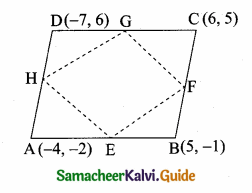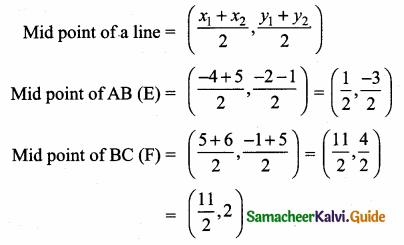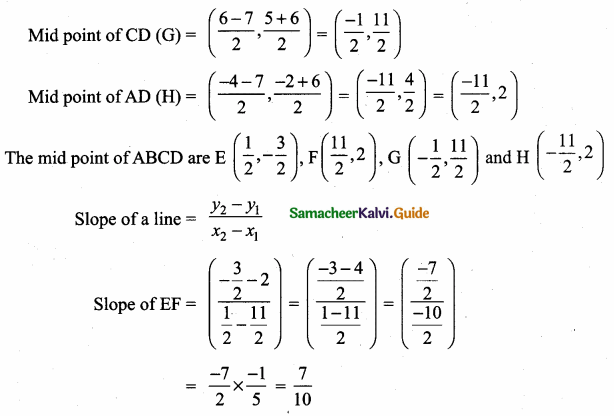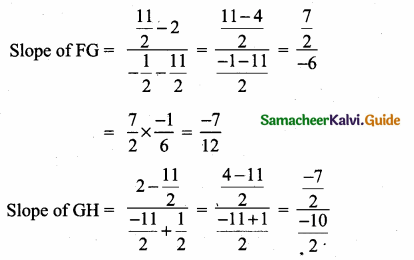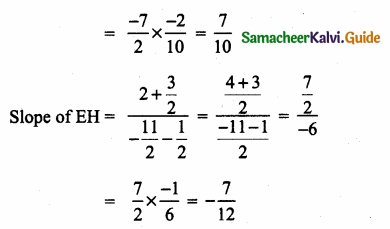Slope of EF = Slope of GH = $$\frac { 7 }{ 10 }$$
∴ EF || GH …….(1)
Slope of FG= Slope of EH = – $$\frac { 7 }{ 12 }$$
∴ FG || EH ……(2)
From (1) and (2) we get EFGH is a parallelogram.
The mid point of the sides of the Quadrilateral ABCD is a Parallelogram.Question 14.
PQRS is a rhombus. Its diagonals PR and QS intersect at the point M and satisfy QS = 2PR. If the coordinates of S and M are (1, 1) and (2, -1) respectively, find the coordinates of P.
Solution:
Slope of a line = $$\frac{y_{2}-y_{1}}{x_{2}-x_{1}}$$
Slope of SM = $$\frac { 1+1 }{ 1-2 }$$ = $$\frac { 2 }{ -1 }$$ = -2
Slope of PM = $$\frac { 1 }{ 2 }$$ (Since SM and PM are ⊥r)
Let the point p be (a,b)
Slope of PM = $$\frac { 1 }{ 2 }$$
$$\frac { b+1 }{ a-2 }$$ = $$\frac { 1 }{ 2 }$$ ⇒ a – 2 = 2b + 2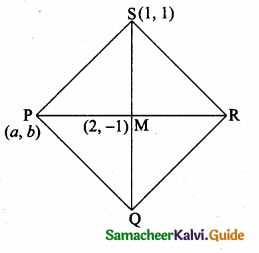a – 2b = 4
a = 4 + 2b ……(1)
Given QS = 2PR
$$\frac { QS }{ 2 }$$ = PR
∴ SM = PR
SM = 2PM (PR = 2PM)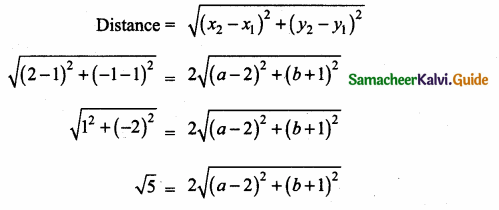Squaring on both sides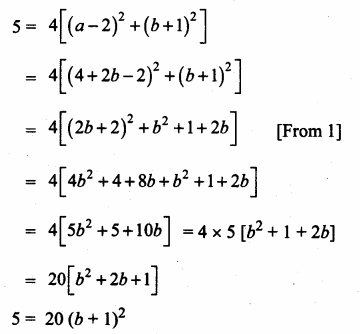∴ (b + 1)2 = $$\frac { 1 }{ 4 }$$ ⇒ b + 1 = ± $$\frac { 1 }{ 2 }$$
b = $$\frac { 1 }{ 2 }$$ – 1 (or) b = – $$\frac { 1 }{ 2 }$$ – 1
= – $$\frac { 1 }{ 2 }$$ – 1 (or) b = –$$\frac { 1 }{ 2 }$$ – 1
= – $$\frac { 1 }{ 2 }$$ (or) – $$\frac { 3 }{ 2 }$$
a = 4 + 2b
a = 4 + 2 ($$\frac { -1 }{ 2 }$$)
a = 3
a = 4 + 2 ($$\frac { -3 }{ 2 }$$)
a = 4 – 3
a = 1
The point of p is (3,$$\frac { -1 }{ 2 }$$) (or) (1,$$\frac { -3 }{ 2 }$$)